# Math help from the Learning Centre

This guide provides useful resources for a wide variety of math topics. It is targeted at students enrolled in a math course or any other Centennial course that requires math knowledge and skills.

## Operations on Signed numbers

Real Numbers are not just out of thin air, but have connections with real life. So, positive numbers like 2, 3, 89, 3/4 make sense immediately. But, what about negative numbers like -2, -4, -5, -100, -1/3? Watch the following video to understand these connections.

Now that we understand from the above video where the signed numbers come from, let's visualize some operations like adding or subtracting of signed numbers. A number line is a very helpful tool.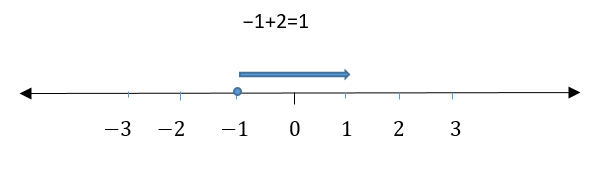Notice when you are adding, no matter which number you start from, you move that many spots to the right. This is the basic understanding of the adding of signed numbers. Notice the right moving arrow. See more examples below.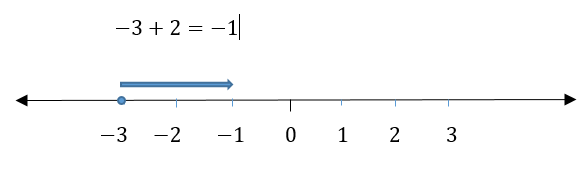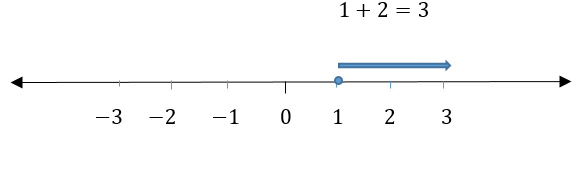Notice when you are subtracting, no matter which number you start from, you move that many spots to the left. This is the basic understanding of the subtracting of signed numbers. Notice the left moving arrow. See examples below.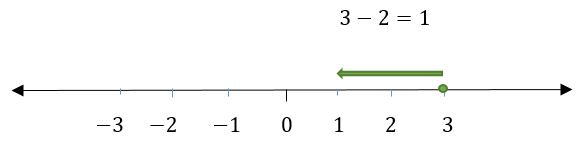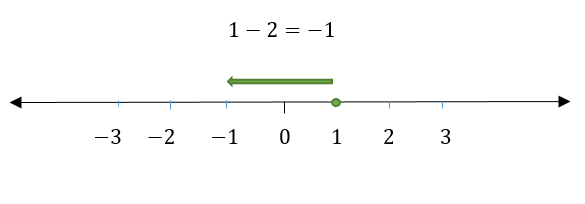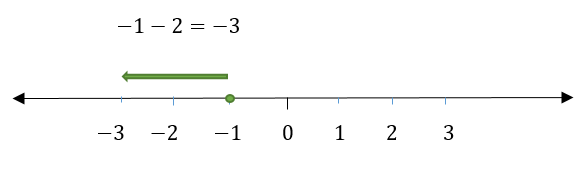Also, realize that visualizing a number line helps in the basic understanding of how these operations work, but it could become cumbersome if working with really large numbers. Hence, please check out the following tips.

## Tips to signed numbers

 Type of Operations between numbers Types Example/Result Addition/Subtraction Adding two positive numbers Always a positive solution, and you add the values. E.g., 4 + 47 = 51 Adding two negative numbers Always a negative solution, and you add the values. E.g., - 4 - 47 = -51 Adding a negative and a positive number A negative solution if the bigger number is a negative signed number, and you subtract the values. E.g., - 47 + 4 = - 43 A positive solution if the bigger number is a positive signed number, and you subtract the values. E.g., - 4 + 47 = 43 Multiplication/Division Multiplying/Dividing two positive numbers Always a positive solution. E.g., 25 X 5 = 125, 25/5 = 5 Multiplying/Dividing two negative numbers Always a positive solution. E.g., (- 25) X (- 5) = 125, (- 25)/ (- 5) = 5 Multiplying/Dividing a negative and a positive number Always a negative solution. E.g., (- 25) X 5 = - 125, (- 25)/5 = - 5,  25 X (- 5) = - 125, 25/ (- 5) = - 5